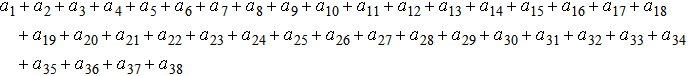prev ( testsuite > Topics > LineBreak > linebreaking014-linebreak4  ) next

Alternatives: (mml file)  (full) (simple) (plain) (form) (slideshow)
File:Topics/LineBreak/linebreaking014-linebreak4
CVS-ID:
Author:Autumn Cuellar, dessci.com
Description:Linebreaking: Linebreak="nobreak" on mspace. Because the mspace is next to an mo, the mspace is effectively a no-op (3.2.7.2) and breaks occur on the '+'s as they would without the mspace being present.

Sample Rendering:${a}_{1}\phantom{\rule{0ex}{0ex}}+{a}_{2}\phantom{\rule{0ex}{0ex}}+{a}_{3}\phantom{\rule{0ex}{0ex}}+{a}_{4}\phantom{\rule{0ex}{0ex}}+{a}_{5}\phantom{\rule{0ex}{0ex}}+{a}_{6}\phantom{\rule{0ex}{0ex}}+{a}_{7}\phantom{\rule{0ex}{0ex}}+{a}_{8}\phantom{\rule{0ex}{0ex}}+{a}_{9}\phantom{\rule{0ex}{0ex}}+{a}_{10}\phantom{\rule{0ex}{0ex}}+{a}_{11}\phantom{\rule{0ex}{0ex}}+{a}_{12}\phantom{\rule{0ex}{0ex}}+{a}_{13}\phantom{\rule{0ex}{0ex}}+{a}_{14}\phantom{\rule{0ex}{0ex}}+{a}_{15}\phantom{\rule{0ex}{0ex}}+{a}_{16}\phantom{\rule{0ex}{0ex}}+{a}_{17}\phantom{\rule{0ex}{0ex}}+{a}_{18}\phantom{\rule{0ex}{0ex}}+{a}_{19}\phantom{\rule{0ex}{0ex}}+{a}_{20}\phantom{\rule{0ex}{0ex}}+{a}_{21}\phantom{\rule{0ex}{0ex}}+{a}_{22}\phantom{\rule{0ex}{0ex}}+{a}_{23}\phantom{\rule{0ex}{0ex}}+{a}_{24}\phantom{\rule{0ex}{0ex}}+{a}_{25}\phantom{\rule{0ex}{0ex}}+{a}_{26}\phantom{\rule{0ex}{0ex}}+{a}_{27}\phantom{\rule{0ex}{0ex}}+{a}_{28}\phantom{\rule{0ex}{0ex}}+{a}_{29}\phantom{\rule{0ex}{0ex}}+{a}_{30}\phantom{\rule{0ex}{0ex}}+{a}_{31}\phantom{\rule{0ex}{0ex}}+{a}_{32}\phantom{\rule{0ex}{0ex}}+{a}_{33}\phantom{\rule{0ex}{0ex}}+{a}_{34}\phantom{\rule{0ex}{0ex}}+{a}_{35}\phantom{\rule{0ex}{0ex}}+{a}_{36}\phantom{\rule{0ex}{0ex}}+{a}_{37}\phantom{\rule{0ex}{0ex}}+{a}_{38}$